# EXPERIMENTAL MODAL ANALYSIS OF ULTRASONIC SURGICAL WAVEGUIDES USING EFFECT OF INVERSE MAGNETOSTRICTION

Experimental modal analysis (EMA) of ultrasonic waveguides is important for many applications including design of ultrasonic surgical tools. However, most of the known sensors and instruments for measuring parameters of ultrasonic vibrations are limited to the measurements on the face surface of the ultrasonic waveguide and therefore unable to determine the spatial distribution of the parameters along its length, which in turn makes it difficult to identify natural modes and shapes. The article describes EMA of ultrasonic surgical waveguides based on the effect of inverse magnetostriction. During vibration of an ultrasonic waveguide the inverse magnetostriction generates alternating magnetic field which can be detected by means of an induction coil. Spatial resolution >of the measurements can be achieved by application of the sensors with flat (spiral) induction coils. The sensors used in the study have simple design and low cost making them affordable for wide circle of scientists and engineers. The results of experimental studies implemented on exemplary waveguide for ultrasonic angioplasty made of AISI 321 steel are presented and discussed. The article also discusses the methods for theoretical and experimental determination of the sensitivity of the sensors used in the study. Experimental data are validated by comparing them to the results of computer-aided modal analysis using the finite element method (FEM) and found to be in good agreement with the results of FEM modeling (relative deviation of the vibratory displacements amplitude is about 4.7 %). As it follows from the experimental data and their comparison to the results of FEM modeling, the effect of inverse magnetostriction can be efficiently used for EMA of ultrasonic waveguides.

Keywords:
modal analysis, ultrasonic waveguide, inductive sensor, inverse magnetostriction

Authors: D. Stepanenko;  V. Minchenya;  K. Bogdanchuk
Authors place of work: Belarussian national technical university, Minsk, Republic of Belarus
Published in the journal: Lékař a technika - Clinician and Technology No. 1, 2015, 45, 10-15
Category: Původní práce

## Summary

Experimental modal analysis (EMA) of ultrasonic waveguides is important for many applications including design of ultrasonic surgical tools. However, most of the known sensors and instruments for measuring parameters of ultrasonic vibrations are limited to the measurements on the face surface of the ultrasonic waveguide and therefore unable to determine the spatial distribution of the parameters along its length, which in turn makes it difficult to identify natural modes and shapes. The article describes EMA of ultrasonic surgical waveguides based on the effect of inverse magnetostriction. During vibration of an ultrasonic waveguide the inverse magnetostriction generates alternating magnetic field which can be detected by means of an induction coil. Spatial resolution >of the measurements can be achieved by application of the sensors with flat (spiral) induction coils. The sensors used in the study have simple design and low cost making them affordable for wide circle of scientists and engineers. The results of experimental studies implemented on exemplary waveguide for ultrasonic angioplasty made of AISI 321 steel are presented and discussed. The article also discusses the methods for theoretical and experimental determination of the sensitivity of the sensors used in the study. Experimental data are validated by comparing them to the results of computer-aided modal analysis using the finite element method (FEM) and found to be in good agreement with the results of FEM modeling (relative deviation of the vibratory displacements amplitude is about 4.7 %). As it follows from the experimental data and their comparison to the results of FEM modeling, the effect of inverse magnetostriction can be efficiently used for EMA of ultrasonic waveguides.

Keywords:
modal analysis, ultrasonic waveguide, inductive sensor, inverse magnetostriction

## Introduction

Experimental modal analysis (EMA) of ultrasonic waveguides is important for many practical applications including design of ultrasonic surgical tools. At present there is a number of sensors and instruments for measuring parameters of ultrasonic vibrations including fiber-optic sensors , laser Doppler vibrometers, inductive sensors [2, 3] and capacitive sensors . Direct measurement of the amplitude of ultrasonic vibrations can be performed by means of a microscope and can be used for calibration of the sensors mentioned above [5; 6]. However, relevant applications of the listed devices known from the literature are limited to the measurements of amplitude on the face surfaces of ultrasonic waveguides and therefore give no possibility to measure its values in intermediate cross-sections of the waveguide. This makes it impossible to measure the spatial distribution of the vibratory parameters along the length of the waveguide and to identify the eigenmodes and natural shapes in detail. Exclusions are some precision inductive sensors capable for registration of very small transverse deformations arising during longitudinal vibrations of ultrasonic waveguides . In this work we shall describe the method of EMA of ultrasonic waveguides based on application of the inductive sensors previously described by the authors in  and relying on the effect of inverse magnetostriction arising in ultrasonic waveguides during their vibrations.

The effect of inverse magnetostriction used for EMA in the present study consists in alteration of the magnetization of ferromagnetic materials under the action of mechanical stresses. When the material is subjected to alternating (cyclic) mechanical stresses, the inverse magnetostriction generates an alternating magnetic field which can be detected by means of an induction coil in the form of electromagnetically induced voltage, a so-called electromotive force (EMF). This method of detection of ultrasonic stresses was for the first time described by Langenecker . Approximately at the same time the possibility of measurement of ultrasonic vibration parameters by means of a voice coil of loudspeaker was described by Garcia . A magnetostrictive rod made of nickel with an induction coil wound on it can be used for determination of the intensity of ultrasonic waves in liquids . The induction coils can also be used for detection of the laser-induced ultrasonic waves in ferromagnetic solids . One of the manifestations of the inverse magnetostriction consists in generation of electromagnetic radiation by means of pulsed focused ultrasound, which can be used for the measurement of electromagnetic properties of materials [11, 12]. Spatial resolution of the measurements in the present study is achieved by application of the sensors with flat (spiral) induction coils. The spatial resolution in this case is determined by the thickness of the induction coil.

The inductive sensors used for implementation of the method considered in this work have simple design and low cost making them affordable for a wide circle of scientists and engineers. Details of design and fabrication technology of the sensors can be found in .

## Methodology and results of the experimental studies

Experimental studies were implemented on multi-stepped waveguides for ultrasonic angioplasty made of AISI 321 stainless steel. The design of the waveguides was described in .

The frequency of vibration of the waveguides was set to the values ensuring the resonance of the longitudinal vibrations. The waveguide was scanned along the length with 2 mm step and the oscillogram of the signal from the sensor was recorded for each position. The position of the sensor relative to the waveguide was measured from the working end (head) of the waveguide. Waveguide during the measurements was inserted into the central opening of the sensor and was enclosed by its coil. The output signal of the sensor had essentially non-harmonic nature Therefore the signal had been analyzed using the discrete Fourier transform.

Fig. 1 presents an example of distribution of the first and the third harmonic components of the signal along the length of the waveguide. The results were obtained for the waveguide with length 235 mm (only portion from 0 to 192 mm is shown in the Fig. 1). The cross-section diameters are changed stepwise along the length: Ø2 mm within 5–35 mm section and Ø0.9 mm within 40–235 mm section. Smooth transitions between the portions with different diameters were provided. The first natural frequency of longitudinal vibrations of the waveguide was experimentally found to be 25155 Hz.

Fig. 1 shows distribution of the signal amplitude for the portion of the waveguide situated between the first and the third antinodes of the vibratory displacements. It shall be shown later that the amplitude of the output signal of the inductive sensor is proportional to the amplitude of internal forces (i.e. mechanical stresses) in the waveguide. As a consequence the antinodes of the vibratory displacements will correspond to the nodes of distribution of the signal amplitude.

Output power of the ultrasonic generator was 45 W. Maximum amplitude of the sensor signal was about 25.4 mV. It is seen from Fig. 1 that the third harmonic of the signal has a spatial wavelength equal to 1/3 of the wavelength of the first harmonic. This means that both harmonics are generated by the longitudinal vibrations with frequency ratio 1:3. Simultaneous existence of longitudinal vibrations with multiple frequencies can be explained by non-linearity of the conversion function of the piezoelectric ultrasonic transducer used for excitation of the vibrations in the waveguide: Langevin-type piezoelectric transducers generate non-harmonic vibratory displacements when high-power driven . Presence of high-order harmonics in the signal spectrum can be also related to non-linearity of the transfer function of the sensor itself. However, in this case non-zero amplitudes of the first harmonic should correspond to non-zero amplitudes of the third harmonic. At the same time in the Fig. 1 some points with non-zero amplitudes of the first harmonic correspond to the nodal points of the third harmonic. This excludes non-linearity of the sensor.Fig. 1: Distribution of the first and third harmonic components of the output signal of inductive sensor along the length of the waveguide. 1 – first harmonic component, 2 – third harmonic component, 3, 4 – steps of the waveguide, 5 – transitional section between steps of the waveguide, 6 – head of the waveguide

## Theory of EMA data processing

Electromotive force (EMF) induced in a single loop of the sensor’s coil can be determined using Faraday-Lenz law: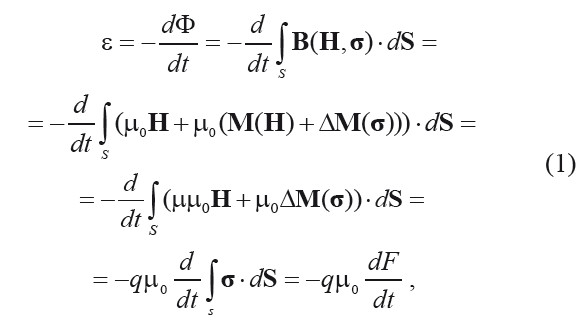where Φ is magnetic flux passing through the loop; S is a vector cross-sectional area of the waveguide; B(H, σ) is the induction of magnetic field inside the material of the waveguide, when mechanical stresses σ and external biasing field with strength H are applied; M(H) is magnetization of unstressed material (σ = 0) at biasing field H; μ0 is the magnetic constant; μ is relative magnetic permeability of the material; q is a coefficient of magneto-mechanical coupling describing the effect of inverse magnetostriction (); ΔM(σ) is alteration of magnetization produced by application of mechanical stresses σ (here, the case of uniaxial stress is assumed); F is a magnitude of internal force.

Eq. (1) takes into account that term μμ0H is stationary. As a consequence its time derivative will take zero value and final result will not depend on magnetic permeability of the material.

When the magnitude F of internal force depends on time harmonically as , where f is a frequency of vibrations, Eq. (1) can be rewritten as follows:It follows from Eq. (2) that amplitude of EMF is proportional to the frequency of vibrations. At substantially large frequencies (20..30 kHz) this enables generation of rather strong signal even at very small alterations of magnetization.

Coefficient q in Eq. (2) is related to magnetostrictive constant d33: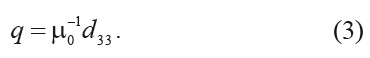This enables determination of the sensor sensitivity for the known value of magnetostrictive constant. Magnetostrictive properties of the waveguide material can be determined by measuring its deformations induced by definite magnetic field using various direct and indirect methods .

Sensitivity of the sensor with respect to internal force is given as follows:where is the amplitude of internal force, is the amplitude of the output voltage of the sensor.

The sensitivity can be calculated from the measurements of stresses in the waveguide using strain gauges for instance . However, measurement of stresses by means of strain gauges is indirect (needs for knowledge of gage factor) and non-local (for improvement of spatial resolution it is necessary to use short-length strain gauges) as well as relatively complicated, which makes this technique not efficient.

In this work we evaluate the sensor sensitivity using the direct optical measurements of the amplitude of vibratory displacements and theoretical relations between stresses and vibratory displacements. When a waveguide is ultrasonically vibrated, the inertia of human vision results in apparent elongation of the marking on the surface of the waveguide. The value of this elongation is equal to doubled amplitude (peak-to-peak value) of the vibratory displacement. Hence, the optical method is based on selection of a control marking on the surface of the waveguide and measurement of its observable length during vibration. Control marking can be a natural marking, e.g. a scratch on the surface, or an artificial marking intentionally created on the surface. The measurements were implemented using microscope with magnification 485x equipped with ocular micrometer screw with magnification 15x and epi-objective with magnification 485/15=32.3x. The smallest scale division of the ocular micrometer screw corresponds to 10 μm displacement in frontal focal plane of the ocular or 10/32.3=0.309 μm displacement in frontal focal plane of the objective (object plane).

Theoretical relation between amplitude of normal mechanical stresses over the cross-section of the waveguide and amplitude of the vibratory displacements along the waveguide is written as follows: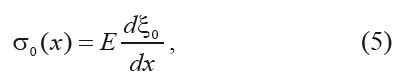where E is elastic modulus of the waveguide material. Here, the case of elastic one-dimensional loading along the axis of the waveguide is assumed. Equation (5) can be used for calculation of the sensor sensitivity.

The amplitude of vibratory displacements can be found from Eq. (5) by means of integration: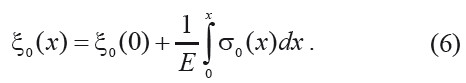Since , where is cross-sectional area of the waveguide, then Eq. (6) can be written as follows:or with account for Eq. (4)The sensitivity can be readily obtained from Eq. (8) if amplitudes and of vibratory displacements for two cross-sections of the waveguide and distribution of output voltage amplitude along the length of the respective part of the waveguide between these cross-sections are known:If cross-sections and are situated at different sides relative to the node of vibratory displacements, then amplitudes and have opposite signs indicating that the vibrations of corresponding cross-sections are antiphase. The sign of amplitudes can be neglected if represent Eq. (9) in the following form: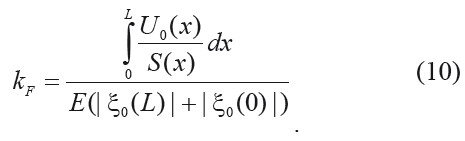Integral in Eq. (10) can be calculated by means of numerical integration of experimental dependence . During integration it is necessary to account for the change of sign of the output voltage amplitude during transition through the nodal points.

Evaluating the distribution of amplitude of vibratory displacements along the waveguide, it is rational to use parameter , which incorporates elastic modulus, instead of sensitivity . This is especially useful, when elastic modulus E is not precisely known. Parameter can be determined from experimental results without explicit use of the value of elastic modulus E:For the known value of parameter distribution can be determined from equationwhich follows from Eq. (8).

Elastic modulus E can be determined from the known value of wavelength λ:where ρ is density of the waveguide material, c is a speed of longitudinal ultrasonic waves in the waveguide material.

Wavelength λ can be found from measured amplitude of the output voltage for the portion of the waveguide with constant cross-sectional area with the length not less than λ/2. Distance between two successive nodes of distribution will be equal to half-wavelength λ/2.

### Experimental application of EMA data processing technique

As initial data for calculation of the sensitivity we used experimental dependence presented in the Fig. 1. We also used values , and of vibratory displacements amplitude in the first, second and third antinodes of the vibratory displacements. The amplitudes of vibratory dis-placements were measured by means of direct optical method and had the following values: , . Calculation of parameter from the values of amplitude measured in the cross-sections and yielded value . Validation of the results was performed by comparing the calculated value of vibratory displacements amplitude in the cross-section with the experimentally measured value for this cross-section (). The calculated value was found to be . Absolute deviation of the calculated value from the experimental one is comparable to the value of the smallest scale division of the ocular micrometer screw (0.309 μm) and corresponds to relative deviation 7.8 %. So, the calculated and the experimental values are in good agreement.

Values of and were found to be 92 and 192 mm and can be used for calculation of the wavelength: . If the density of the waveguide material is and the frequency of vibrations is , then the calculated value of elastic modulus is and the calculated value of the speed of sound is . For comparison: tabular value of the elastic modulus for AISI 321 steel is 196 GPa. Value of the sensor sensitivity was found to be .

## Validation of the experimental results

The experimental results were validated by comparing them to the data obtained by means of finite element method (FEM) using ANSYS software. Essentially the natural frequencies and the shapes of longitudinal vibrations were considered.

Calculated natural frequency was found to be 26609 Hz (experimental value is 25155 Hz, relative deviation is 5.8 %). Fig. 2 presents distributions of vibratory displacements amplitude along the length of the waveguide calculated using FEM (curve No. 1) and obtained from experimental data using Eq. (12) (curve No. 2). The amplitude is expressed in relative (arbitrary) units (a.u.), i.e. absolute values of amplitude are normalized to the value of the vibratory displacements amplitude at the end of the waveguide.

Mean-square deviation of the results obtained by means of FEM from the results calculated using Eq. (12) was found to be about 0.047 a.u. (i.e. about 4.7 % from maximum value of amplitude).

Absolute error in determination of coordinate x0 of nodal plane is 3.1 mm (calculation from Eq. (12) gives x0 = 41.0 mm, calculation using FEM gives x0 = 44.1 mm), relative error is 7.6 %. Gain factor calculated using the vibration amplitudes obtained from Eq. (12) is 4.849 μm/2.1 μm = 2.309, gain factor calculated using FEM data is 2.464 (relative error is 6.7 %).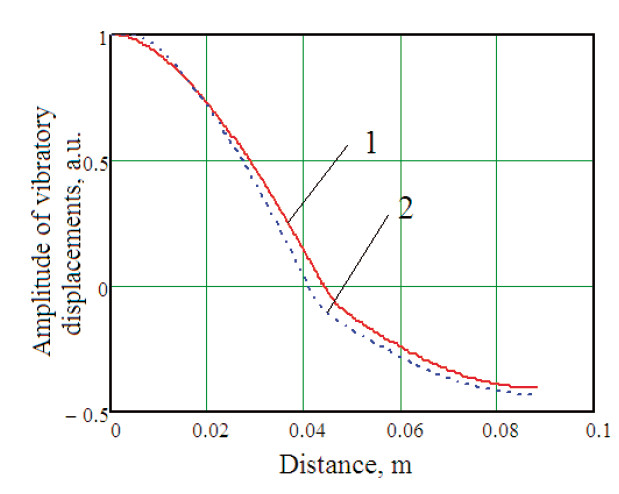Fig. 2: Distribution of vibratory displacements amplitude along the length of the waveguide. 1 – calculation using FEM, 2 – calculation from experimental data

It can be seen from the data presented that the main parameters of the waveguide vibration determined using FEM and obtained using the proposed experimental method match with rather good precision. Hence, the formalism presented in this work can be used for development of the sensory systems for evaluation of the waveguide performance.

## Conclusions

It is shown that the effect of inverse magnetostriction can be efficiently used for experimental modal analysis of ultrasonic waveguides of complex geometry.

The method of experimental evaluation of the sensitivity of the sensors based on the effect of inverse magnetostriction is proposed. The method is valid for the ultrasonic applications with substantially large excitation frequencies (20..30 kHz) whereas the resonance of the waveguide longitudinal vibrations should be assured. No explicit elastic modulus data for the waveguide material is necessary for the application of the formalism presented in the work.

The results of the work were validated by comparison to the data obtained using FEM modeling.

Due to simple design and low cost the considered sensors can be affordable for wide circle of scientists and engineers. The method discussed in this work can find wide application in experimental studies and practical design of new types of ultrasonic waveguides for medical and industrial applications.

Dmitry Stepanenko, Ph.D.

Department of construction

and production of instruments

Instrument-making faculty

Belarussian national technical university

65 Nezavisimosty Ave.,

220027 Minsk, Republic of Belarus

E-mail: stepd@tut.by

Phone: +375 172 939 101

##### Zdroje

 Displacement measurement principle using fiber optic sensors – an introduction to the Fotonic Sensor. http://www.mtiinstruments.com/products/fiberopticmeasurement.aspx

 Solomakho, D., Minchenya, V. Application of noncontact inductive sensors for the purpose of flexible thrombolysis waveguides acoustic parameters control. Proc. of the 51st International scientific colloquium “Information technology and electrical engineering – devices and systems, materials and technologies for the future” [CD-ROM], Ilmenau, 2006, ISBN 3-938843-16-0.

 Stepanenko, D. et al. Induction sensors for measurement of vibration parameters of ultrasonic surgical waveguides. Lékař a Technika, 2012, vol. 42, No. 2, p. 11-14.

 Lanin, V. L. et al. Instrumental ensuring of measurement of parameters of ultrasonic effects in technological processes // Technology and design in electronic equipment (Tehnologija i konstruirovanie v jelektronnoj apparature), 2008, No. 2, p. 51-55. (in Russian).

 Abramenko, D. S. Methods and means for control of vibration amplitude of piezoelectric transducers of ultrasonic technological devices: synopsis of PhD dissertation. – Barnaul, 2010. – 23 p. (in Russian).

 Volkov, S. S. et al. Welding of plastics with ultrasound. – Moscow, 1974. – 264 p. (in Russian).

 Langenecker, B. Inverse magnetostrictive effect of ultrasonic stress waves in steel. Journal of Acoustical Society of America, 1965, vol. 38, p. 234-238.

 Garcia, R., Hammitt, F. G. Amplitude determination of an ultrasonic transducer by means of an accelerometer assembly: Internal report 05031-7-I. – The University of Michigan, Laboratory for Fluid Flow and Heat Transport Phenomena, 1965. – 21 p.

 Smith, A. W., Weimer, D. K. Comparison of supersonic intensities by means of a magnetostriction gauge. Review of Scientific Instruments, 1947, vol. 18, p. 188-190.

 Kozhushko, V. V. et al. Detection of broadband laser induced longitudinal ultrasonic pulses in ultrafine grain nickel by pancake coil. AIP Conference Proceedings, 2012, vol. 1433, p. 733-736.

 Takashima, K. et al. Nondestructive inspection of austenitic stainless steels by detection of acoustically stimulated electromagnetic response. Proc. of Symposium on Ultrasonic Electronics, 2011, vol. 32, p. 349-350.

 Yamada, H. et al. Magnetic imaging by ultrasonic techniques. Proc. of Symposium on Ultrasonic Electronics, 2009, vol. 30, p. 57-58.

 Stepanenko, D. A., Minchenya, V. T. Modeling of flexible waveguides for ultrasonic vibrations transmission: longitudinal and flexural vibrations of non-deformed waveguide. Ultrasonics, 2010, vol. 50, p. 424-430.

 Guyomar, D. et al. Piezoelectric ceramics nonlinear behavior. Application to Langevin transducer. Journal de Physique III France, 1997, vol. 7, p. 1197-1208.

 Chechernikov, V.I. Magnetic measurements. – Moscow, 1969. – 385 p. (in Russian).

 Lamport, H., Zinsser, H.H. Strain gauge measurement of output of magnetostrictive ultrasonic transducer – pitfalls of optical measurement. Journal of Acoustical Society of America, 1950, vol. 31, p. 435-438.

##### Štítky
Biomedicína

Článek vyšel v časopise

### Lékař a technika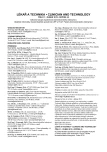2015 Číslo 1

Nejčtenější v tomto čísle
Kurzy

Zvyšte si kvalifikaci online z pohodlí domova##### Efektivní snižování hyperpigmentace − od výzkumu k inovacím pro praxi
nový kurz
Autoři: dr. Ludger Kolbe, dr. Thierry Passeron, MD, PhD

##### Postavení moxonidinu a indapamidu v léčbě hypertenze
Autoři: MUDr. Jan Vachek

##### Magnetická rezonance a diagnostika axiálních spondyloartritid - Virtuální trénink
Autoři: MUDr. Leona Procházková, Ph.D., MUDr. Monika Gregová, Ph.D., MUDr. Vladimír Červeňák, MUDr. Eva Korčáková, Ph.D.

##### Následná péče po prodělaném covid-19
Autoři:

Všechny kurzy
Přihlášení
Zapomenuté heslo

Nemáte účet?  Registrujte se

Zapomenuté heslo

Zadejte e-mailovou adresu, se kterou jste vytvářel(a) účet, budou Vám na ni zaslány informace k nastavení nového hesla.

Přihlášení

Nemáte účet?  Registrujte se